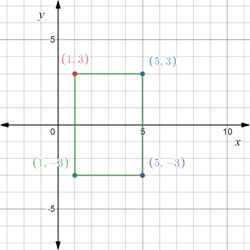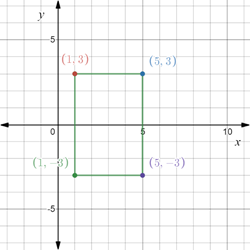# The area of the rectangle with vertices A ( 1 , 3 ) , B ( 5 , 3 ) , C ( 1 , − 3 ) and D ( 5 , − 3 ) and also plot the vertices on the coordinate plane.### Precalculus: Mathematics for Calcu...

6th Edition
Stewart + 5 others
Publisher: Cengage Learning
ISBN: 9780840068071### Precalculus: Mathematics for Calcu...

6th Edition
Stewart + 5 others
Publisher: Cengage Learning
ISBN: 9780840068071

#### Solutions

Chapter 1.8, Problem 19E
To determine

## To calculate: The area of the rectangle with vertices A(1,3),B(5,3),C(1,−3) and D(5,−3) and also plot the vertices on the coordinate plane.

Expert Solution

The area of the rectangle is 24 sq units . The rectangle so formed is provided below,### Explanation of Solution

Given information:

The vertices of rectangle A(1,3),B(5,3),C(1,3) and D(5,3) .

Formula used:

Distance d(A,B) between two points A=(x1,y1) and B=(x2,y2) in the Cartesian plane is denoted by d(A,B)=(x2x1)2+(y2y1)2 .

Area of rectangle is l×b where l is the length and b is the breadth of the rectangle.

Calculation:

Consider the vertices of rectangle A(1,3),B(5,3),C(1,3) and D(5,3) .

Toplot the points A(1,3),B(5,3),C(1,3) and D(5,3) in the coordinate plane.

Construct a Cartesian plane with vertical axis as y-axis and horizontal axis as x-axis.

Marks the numbers on both the axes.

Plot the points A(1,3),B(5,3),C(1,3) and D(5,3) .

And join them to form a rectangle.Recall that area of rectangle is l×b where l is the length and b is the breadth of the rectangle.

Evaluate the length and breadth of the rectangle obtained above.

Recall that the distance d(A,B) between two points A=(x1,y1) and B=(x2,y2) in the Cartesian plane is denoted by d(A,B)=(x2x1)2+(y2y1)2 .

Evaluate the distance between A(1,3) and B(5,3) .

d(A,B)=(51)2+(33)2=16=4

Evaluate the distance between A(1,3) and C(1,3) .

d(A,C)=(11)2+(33)2=36=6

Therefore, length is 6 and breadth is 4 units.

Now, area of rectangle is,

l×b=6×4=24

Thus, the area of the rectangle is 24 sq units .

### Have a homework question?

Subscribe to bartleby learn! Ask subject matter experts 30 homework questions each month. Plus, you’ll have access to millions of step-by-step textbook answers!